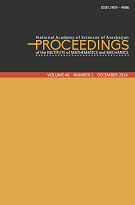﻿ Volume 47, Issue 2 — Proc. Inst. Math. Mech.P R O C E E D I N G S
of the
Institute of Mathematics and Mechanics

 ISSN 2409-4986 (Print) ISSN 2409-4994 (Online)

## Volume 47, Issue 2

Converse theorem of the approximation theory of functions in Morrey-Smirnov classes related to the derivatives of functions

Scalability of \$G\$-frames by diagonal operators

On the error of approximation by RBF neural networks with two hidden nodes
by Arzu M-B. Babayev and Ibrahim K. Maharov

Nonlinear wave equations with nonlinear transmission acoustic condition
by Sevda E. Isayeva

On integration of the loaded Korteweg-de Vries equation in the class of rapidly decreasing functions
by Aknazar B. Hasanov and Umid A. Hoitmetov

On an inverse problem for a parabolic equation in a domain with moving boundaries
by Adalat Ya. Akhundov and Arasta Sh. Habibova

A study of harmonic sections of tangent bundles with vertically rescaled Berger-type deformed Sasaki metric
by Abderrahim Zagane

Calderón-Zygmund operators with kernels of Dini's type and their multilinear commutators on generalized variable exponent Morrey spaces
by Vagif S. Guliyev and Afag F. Ismayilova

The well-posedness of the mixed problem for one system of thermoelasticity with a singular coefficient
by Akbar B. Aliyev and Gunay R. Gadirova

Solvability of a boundary value problem for a second order elliptic differential-operator equation with a complex parameter
by Bahram A. Aliev, Vugar Z. Kerimov and Yakov S. Yakubov

\$\Gamma\$-rings of weak cosets in nearness approximation spaces
by Ebubekir Inan and Mustafa Uckun

On an inverse problem for the system of Dirac equations with discontinuity conditions on the whole axis
by Gulshan M. Azimova and Hidayat M. Huseynov

Global bifurcation from intervals in nonlinear Sturm-Liouville problem with indefinite weight function
by Leyla V. Nasirova

 © 2023 Institute of Mathematics and Mechanics